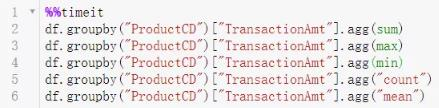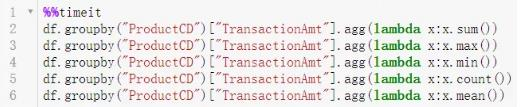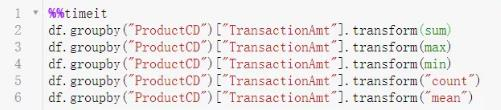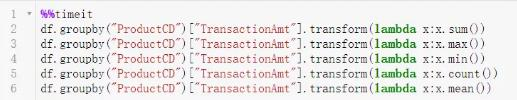# 原创 如何提高Pandas的运行速度？四大性能优化方法

Pandas作为数据分析的屠龙宝刀，毫不夸张的说，功能和优势都极其强大。像是支持GB数据处理，多样的数据清洗方法；支持多种开源可视化工具包，更加丰富的数据成果展示等等。因此如果能做好性能优化，就可以极大的提高Pandas的运行速度。本文为大家总结了四大优化Pandas性能的方法，感兴趣的朋友就赶紧看下去吧！1、数据读取的优化

import pandas as pd

#读取csv

#pkl格式

df.to_pickle('xxx.pkl') #格式另存

#hdf格式

df.to_hdf('xxx.hdf','df') #格式另存

2、进行聚合操作时的优化

在使用 agg 和 transform 进行操作时，尽量使用Python的内置函数，能够提高运行效率。（数据用的还是上面的测试用例

（1）agg+Python内置函数（2）agg+非内置函数可以看到对 agg 方法，使用内置函数时运行效率提升了60%。

（3）transform+Python内置函数（4）transform+非内置函数对 transform 方法而言，使用内置函数时运行效率提升了两倍。

3、对数据进行逐行操作时的优化

假设我们现在有这样一个电力消耗数据集，以及对应时段的电费价格。数据集记录着每小时的电力消耗，如第一行代表2001年1月13日零点消耗了0.586kwh的电。不同使用时段的电费价格不一样，我们现在的目的是求出总的电费，那么就需要将对应时段的单位电费×消耗电量。下面给出了三种写法，我们分别测试这三种处理方式，对比一下这三种写法有什么不同，代码效率上有什么差异。

#编写求得相应结果的函数

def get_cost(kwh, hour):

if 0 <= hour < 7:

rate = 0.6

elif 7 <= hour < 17:

rate = 0.68

elif 17 <= hour < 24:

rate = 0.75

else:

raise ValueError(f'Invalid hour: {hour}')

return rate * kwh

#方法一：简单循环

def loop(df):

cost_list = []

for i in range(len(df)):

energy_used = df.iloc[i]['energy_kwh']

hour = df.iloc[i]['date_time'].hour

energy_cost = get_cost(energy_used, hour)

cost_list.append(energy_cost)

df['cost'] = cost_list

#方法二：apply方法

def apply_method(df):

df['cost'] = df.apply(

lambda row: get_cost(

kwh=row['energy_kwh'],

hour=row['date_time'].hour),

axis=1)

#方法三：采用isin筛选出各时段，分段处理

df.set_index('date_time', inplace=True)

def isin_method(df):

peak_hours = df.index.hour.isin(range(17, 24))

simple_hours = df.index.hour.isin(range(7, 17))

off_peak_hours = df.index.hour.isin(range(0, 7))

df.loc[peak_hours, 'cost'] = df.loc[peak_hours, 'energy_kwh'] * 0.75

df.loc[simple_hours,'cost'] = df.loc[simple_hours, 'energy_kwh'] * 0.68

df.loc[off_peak_hours,'cost'] = df.loc[off_peak_hours, 'energy_kwh'] * 0.6

测试结果：

可以看到，采用 isin() 筛选出对应数据后分开计算的速度是简单循环的近606倍，这并不是说 isin() 有多厉害，方法三速度快是因为它采用了向量化的数据处理方式（这里的isin() 是其中一种方式，还有其他方式，大家可以尝试一下） ，这才是重点。

4、使用numba进行加速

如果在你的数据处理过程涉及到了大量的数值计算，那么使用numba可以大大加快代码的运行效率，numba使用起来也很简单，下面给大家演示一下。（代码处理不具有实际意义，只是展示一下效果）

首先需要安装numba模块

>>>pip install numba

我们用一个简单的例子测试一下numba的提速效果

import numba

@numba.vectorize

def f_with_numba(x):

return x * 2

def f_without_numba(x):

return x * 2

#方法一：apply逐行操作

df["double_energy"] = df.energy_kwh.apply(f_without_numba)

#方法二：向量化运行

df["double_energy"] = df.energy_kwh*2

#方法三：运用numba加速

#需要以numpy数组的形式传入

#否则会报错

df["double_energy"] = f_with_numba(df.energy_kwh.to_numpy())

从测试结果来看，再次凸显出向量化处理的优势，同时numba对原本速度已经很快的向量化处理也能提高一倍多的效率。更多numba的使用方法请参考numba的使用文档。

以上就是提高Pandas运行速度的优化方法，大家都get到了吗？如果想要学习Pandas的实战教程，可以上博学谷官网在线学习《数据分析进阶必备技能：Pandas》课程，想要了解课程的更多内容，可以点击课程链接http://www.openxlive.net/promote/detail-1480.html

### 最新文章4887铁算结果开奖结果小说